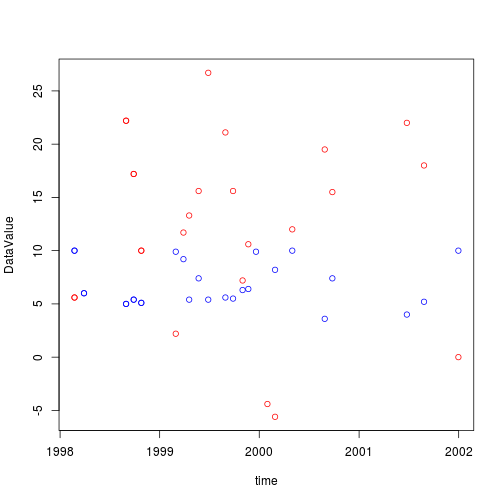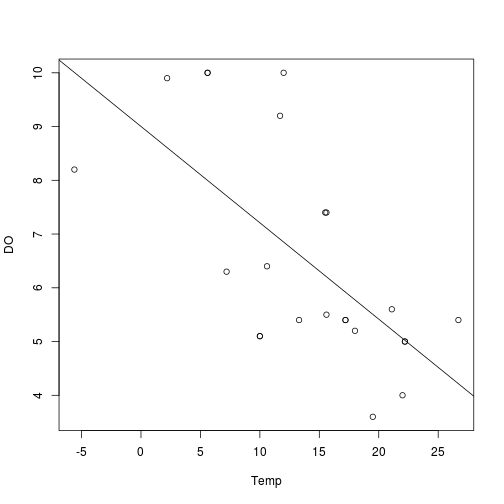This tutorial introduces the WaterML R package. This tutorial shows an example how to retrieve data from the Hydrologic Information System and do statistical analysis in R.

## Data Access Using the WaterML R Package

1. Load the required libraries: WaterML for accessing CUAHSI HIS data. The package can be found in the R CRAN package repository
``````#import required libraries
library(WaterML)
``````
1. Find the CUAHSI HIS services from the HIS Central catalogue. The list of available services registered in HIS Central is also published here: http://hiscentral.cuahsi.org/pub_services.aspx. The GetServices() function returns a table with the URL, description, and citation of each service.
``````#get the list of supported CUAHSI HIS services
services <- GetServices()
``````
``````View(services)
``````
1. Define the CUAHSI HIS service that you are connecting to by giving the URL to that service’s WSDL file. This example uses a service from the Ipswich River Watershed Association: `http://hydroportal.cuahsi.org/ipswich/cuahsi_1_1.asmx?WSDL` that enlists volunteers to collect data on the health of the Ipswich River and its tributaries in Massachusetts, USA. We can use the `GetVariables()` and `GetSites()` functions to get the tables of variables and sites on the server.
``````#point to an CUAHSI HIS service and get a list of the variables and sites
server <- "http://hydroportal.cuahsi.org/ipswich/cuahsi_1_1.asmx?WSDL"
variables <- GetVariables(server)
sites <- GetSites(server)
``````
1. Next we will select one site and find which variables are measured at that site. In this example we choose the site “Fish Brook, Brookview Rd, Boxford” with the full site code “IRWA:FB-BV”. Note that you can learn more about the variables at this site viewing the SiteInfo data table in RStudio.
``````#get full site info for all sites using the GetSiteInfo method
siteinfo <- GetSiteInfo(server, "IRWA:FB-BV")
``````
``````View(siteinfo)
``````
1. Now we will get the data values using the GetValues method for two variables at the site: water temperature (full variable code `IRWA:Temp`) and dissolved oxygen (full variable code `IRWA:DO`). In this example we get the values for all available days. Note that we can also use the `startDate` and `endDate` parameters to restrict the time period of interest. To get help on the GetValues function, you can type `?GetValues` in the R console. Note that for this particular site there are 21 Temperature and 22 dissolved oxygen observations.
``````#get full site info for all sites using the GetSiteInfo method
Temp <- GetValues(server,siteCode="IRWA:FB-BV",variableCode="IRWA:Temp")
DO <- GetValues(server, siteCode="IRWA:FB-BV",variableCode="IRWA:DO")
``````

## Data Analysis and Visualization Using R

1. Plot the time series of temperature and dissolved oxygen. We use the plot function for the new water temperature plot and we use the `points()` function for adding the dissolved oxygen data points to the existing plot.
``````plot(DataValue~time, data=Temp, col="red")
points(DataValue~time, data=DO, col="blue")
``````Note that the “time” represents the local time, and “DateTimeUTC” represents the UTC time. The “DateTimeUTC” columns are in POSIXct format. POSIXct is a special format in R for storing date and time. POSIXct represents the number of seconds since the beginning of 1970. You can use the strftime function to get the year, month, day, hour, minute and second corresponding to each time as shown below:

``````years <- strftime(DO\$time, "%Y")
months <- strftime(DO\$time, "%m")
days <- strftime(DO\$time, "%d")
hours <- strftime(DO\$time, "%h")
minutes <- strftime(DO\$time, "%M")
seconds <- strftime(DO\$time, "%s")
``````
1. Create a merged table with columns: Time, DO (Dissolved Oxygen) and Temp (Temperature). We can create this table using the merge function based on the time column. Note that we renamed the automatically assigned column names in the merged table from DataValue.x to “DO” and from DataValue.y to “Temp”.
``````#merge our two tables based on the time column
data <- merge(DO, Temp, by="time")
#rename the column DataValue.x in the merged table to "DO"
names(data)[names(data)=="DataValue.x"] <- "DO"
#rename the column DataValue.y in the merged table to "Temp"
names(data)[names(data)=="DataValue.y"] <- "Temp"
``````
1. Now you can plot the data as scatter plot of dissolved oxygen concentration versus temperature.
``````plot(DO~Temp, data=data)
``````
1. Finally, we can fit a linear regression model to see is there is a relationship between water temperature and dissolved oxygen concentration at this site.
``````# Perform a linear regression on the dissolved oxygen vs. temperature values
model <- lm(DO~Temp, data=data)
``````
``````summary(model)
abline(model)
``````

## Results

The code creates two outputs when run in RStudio. First, it creates a scatter plot of dissolved oxygen concentration versus water temperature with the linear regression line.Second, it outputs the results from the regression analysis. From these results, there appears to be a significant negative linear relationship between water temperature and dissolved oxygen at this site.

``````#>
#> Call:
#> lm(formula = DO ~ Temp, data = data)
#>
#> Residuals:
#>     Min      1Q  Median      3Q     Max
#> -2.1091 -1.1377 -0.5184  1.1897  3.1494
#>
#> Coefficients:
#>             Estimate Std. Error t value Pr(>|t|)
#> (Intercept)  9.00177    0.69022  13.042 1.54e-11 ***
#> Temp        -0.17927    0.04435  -4.042 0.000587 ***
#> ---
#> Signif. codes:  0 '***' 0.001 '**' 0.01 '*' 0.05 '.' 0.1 ' ' 1
#>
#> Residual standard error: 1.566 on 21 degrees of freedom
#> Multiple R-squared:  0.4376, Adjusted R-squared:  0.4108
#> F-statistic: 16.34 on 1 and 21 DF,  p-value: 0.0005871
``````

## Summary

This tutorial shows how you can use the WaterML library in R to access data from a CUAHSI HIS web service directly within R without the need to first download data to your local computer. While this was demonstrated for a data service hosted by Ipswich River Watershed Association, the WaterML R package can be used to access data from any compliant CUAHSI HIS web service including the 100+ data services listed on the HIS Central website.